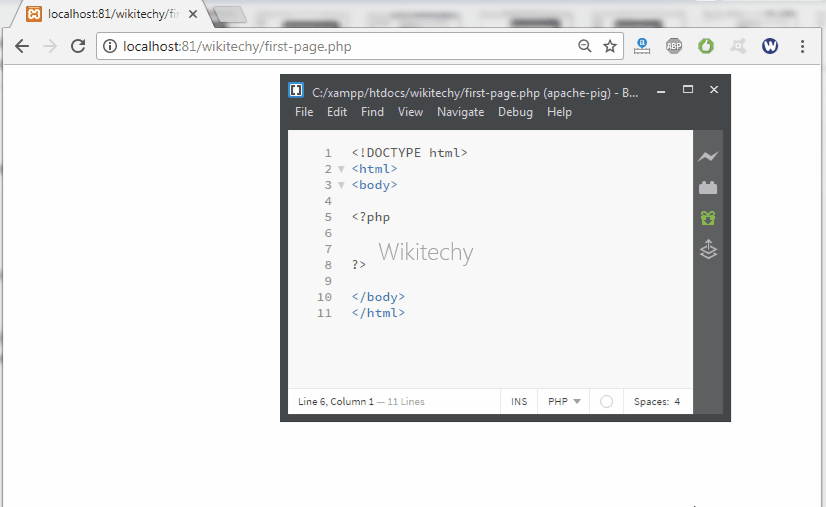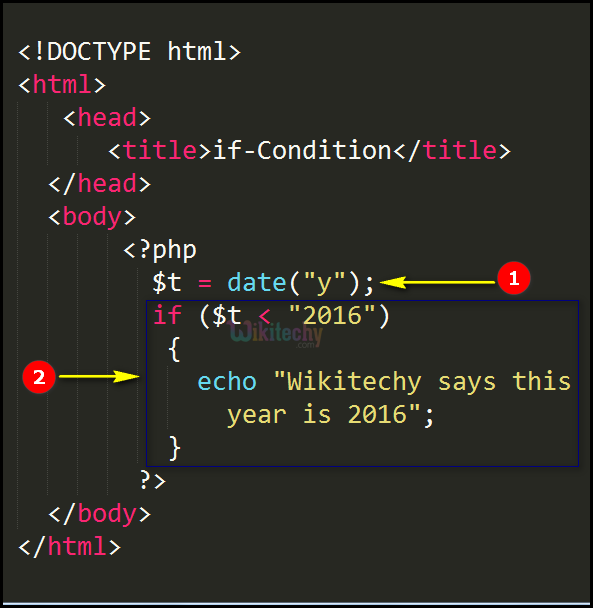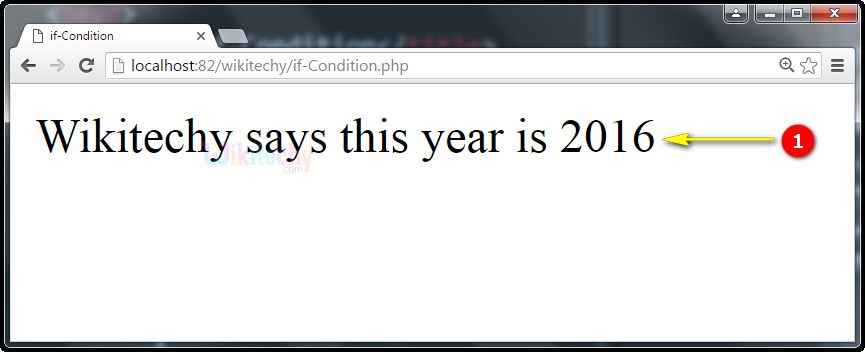# php tutorial - PHP if Condition - php programming - learn php - php code - php script

• The php “if condition “specifies the if expression in terms of Boolean value.
• If the expression evaluates to be TRUE, PHP will execute its statement.Learn PHP - PHP tutorial - If Statement - PHP examples - PHP programs

## php programming Syntax :## php for beginners Code Explanation :1. In PHP, \$t = date(‘’y”) is a variable that defines the Year (Note: In similar way date(“h”) defines hours, date(“w”) defines week).
2. Here, if (\$t > “2016”) expression specifies the current year is less than 2016 condition, which executes the PHP statement” “Wikitechy says this year is 2016”.

## php coding Sample Output :1. Here the PHP statement executes the if condition by printing the echo statement “Wikitechy says this year is 2016”.